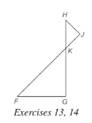Chapter 5.2, Problem 14E### Elementary Geometry for College St...

6th Edition
Daniel C. Alexander + 1 other
ISBN: 9781285195698

#### Solutions

Chapter
Section### Elementary Geometry for College St...

6th Edition
Daniel C. Alexander + 1 other
ISBN: 9781285195698
Textbook Problem
3 views

#If the drawing, △ H J K ∼ △ F G K . If H K = 6 , K F = 8 , and F G = 5 , find H J .

To determine

To calculate: The value of HJ.

Explanation

Given:

ΔHJK~ΔFGK.

HK=6,KF=8 and FG=5.

Approach:

Similarity between Two polygons:

Two polygons are similar if and only if when all pairs of corresponding angles are congruent and all pairs of corresponding sides are proportional.

Calculation:

According to the definition of similar polygon, FG¯HJ¯ and KF¯KH¯

So,

### Still sussing out bartleby?

Check out a sample textbook solution.

See a sample solution

#### The Solution to Your Study Problems

Bartleby provides explanations to thousands of textbook problems written by our experts, many with advanced degrees!

Get Started

#### In Exercises 33-38, rewrite the expression using positive exponents only. 35. x1/3x1/2

Applied Calculus for the Managerial, Life, and Social Sciences: A Brief Approach

#### In Exercises 7-10, Solve the equation. x3x=0

Calculus: An Applied Approach (MindTap Course List)

#### True or False: If f(c) exists and f has a local minimum at c, then f(c) = 0.

Study Guide for Stewart's Single Variable Calculus: Early Transcendentals, 8th